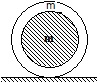#### A thin hollow sphere of mass m is completely filled with an ideal liquid of mass m. When the sphere rolls with a velocity v kinetic energy of the system is equal toOption 1) (1/2)Option 2)Option 3) (4/3)Option 4) (4/5)As we discussed in concept

Kinetic energy of rotation -- wherein= moment of inertia about axis of rotation= angular velocityK.E of the systemOption 1)

(1/2)This is incorrect option

Option 2)This is incorrect option

Option 3)

(4/3)This is correct option

Option 4)

(4/5)This is incorrect option